Welcome to Wall Street Prep! Use code at checkout for 15% off.# Equity Value

Guide to Understanding Equity Value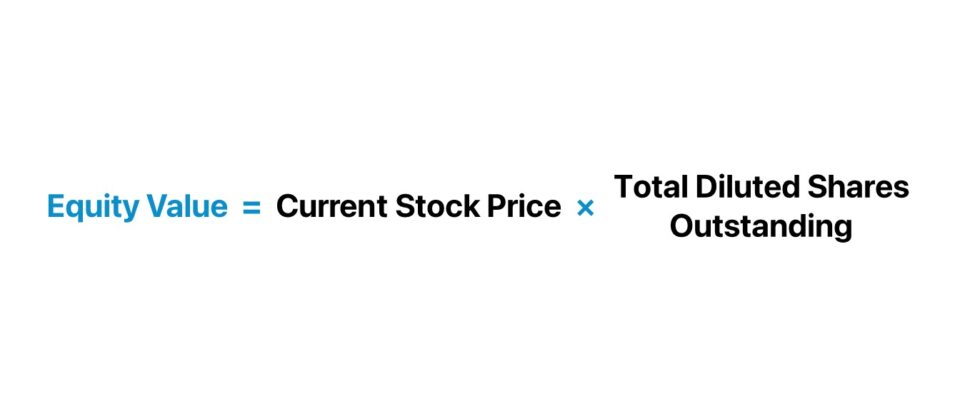• The equity value is the market value of a company’s common equity from the perspective of its shareholders, since all non-equity obligations are neglected.
• The equity value is calculated by multiplying a company’s latest closing stock price by its total number of common shares outstanding on a fully-diluted basis.
• Unlike the enterprise value, the equity value is impacted by the funding sources of the company’s operations (i.e. the capital structure, inclusive of all stakeholders).
• The more debt in the total capitalization of the company, the less residual value remaining for the shareholders with an equity interest – all else being equal.

## What is the Definition of Equity Value?

The equity value is the total value of a company’s common equity from the perspective of its shareholders, as of the latest closing date of the markets.

By measuring the value of a company’s common equity, a practitioner can analyze the current valuation of its total shares outstanding on a diluted basis.

The distinct trait of the equity value metric is that only the value of the common equity belonging to the company is measured, rather than the interests of all capital sources, namely debt, the other source of funding that corporations are most reliant on.

The equity value is the fair market value (FMV) of a company’s common equity at present.

• Market Value of Equity → The equity value, or “market cap”, of a company constantly fluctuates based on its stock price movements and current investor sentiment regarding the underlying issuer (and the financial markets or economic conditions).
• Book Value of Equity (BVE) → In contrast, the book value of equity (BVE), or “Shareholders’ Equity” line item on the balance sheet, represents the value of a company’s common equity prepared for bookkeeping purposes.

Contrary to the market value of equity, the book value of equity (BVE) represents the amount received by common shareholders in the event of default, if all assets belonging to the company were hypothetically liquidated.

Shareholders’ Equity = Total Assets Total Liabilities

Therefore, the equity value refers to the market value of equity and does not refer to the book value of equity. In fact, the variance between the two metrics is substantial for practically all companies, barring unusual circumstances.

## How to Calculate Equity Value?

The company’s current stock price is multiplied by its total common shares outstanding to calculate its equity value.

The shares outstanding component must be expressed on a fully-diluted basis to ensure that any potentially dilutive securities, such as options, warrants, and convertible debt, are taken into account.

The step-by-step process to calculate the equity value is as follows.

1. Determine the Total Number of Basic Shares Outstanding and Current Stock Price as of the Latest Closing Date
2. Estimate the Total Number of Diluted Shares Outstanding Using the Treasury Stock Method (TSM)
3. Multiply the Current Stock Price by the Total Number of Diluted Shares Outstanding to Calculate Equity Value

## Equity Value Formula

The formula used to calculate equity value for publicly traded companies multiplies the latest closing stock price of a company by its total number of diluted shares outstanding.

Equity Value = Latest Closing Stock Price × Total Diluted Shares Outstanding

Where:

• Latest Closing Stock Price → The latest closing stock price is readily available online through sources such as Bloomberg or related media organizations like the Wall Street Journal (WSJ) and CNBC that track and publish share price movements in real-time.
• Total Diluted Shares Outstanding → The process of obtaining the total number of diluted shares outstanding, consists of manually computing the total number of shares outstanding via the Treasury Stock Method (TSM).

The decision to neglect a company’s potentially dilutive securities – i.e. the securities that could be converted into common shares and dilute the common equity component of the capital structure, such as options, warrants, and convertible debt – would be a costly mistake, since those instruments pose a risk to existing shareholders.

## Equity Value vs. Enterprise Value: What is the Difference?

The two metrics, equity value and enterprise value, are the two most common methods to measure the valuation of a company.

• Equity Value → The equity value, or market capitalization (“market cap”), is the total value of a company’s common shares outstanding to its equity holders. The current equity value of a company represents the value of a company’s common equity as of its latest closing date, expressed on a fully diluted basis.
• Enterprise Value (TEV) → The enterprise value is the value of a company’s core operations (i.e. the net operating assets) to all capital providers, such as common equity shareholders, preferred equity holders, and lenders that provided debt financing.

The distinction between enterprise value and equity value is the perspective of the practitioner conducting the analysis itself.

Why? The stake, or vested interest in a company is different for each contributor of capital.

To calculate equity value starting from enterprise value, the process involves removing the value of non-equity claims on the company.

Equity Value = Enterprise Value  Net Debt  Preferred Stock  Minority Interest

Where:

Conceptually, the enterprise value to equity value bridge isolates the residual value of a company belonging to solely shareholders in the company’s common equity.

Since the interests of all stakeholders are represented in enterprise value, the metric is considered capital structure neutral and indifferent to discretionary decisions of management, such as financing decisions (i.e. the percent reliance on debt vs. equity capital).

Hence, the enterprise value is frequently used to perform relative valuation (“comps analysis”), whereas the equity value is not as commonly used for valuation multiples.

On the subject of relative valuation, a fundamental principle in valuation multiples is that for the ratios between a measure of value and a profit metric, the stakeholder(s) reflected in the numerator and denominator must match.

• Enterprise Value MultiplesEV/EBITDA, EV/EBIT, EV/NOPAT, EV/FCFF
• Equity Value MultiplesP/E (Price-to-Equity), P/B (Price-to-Book), PEG Ratio, P/FCFE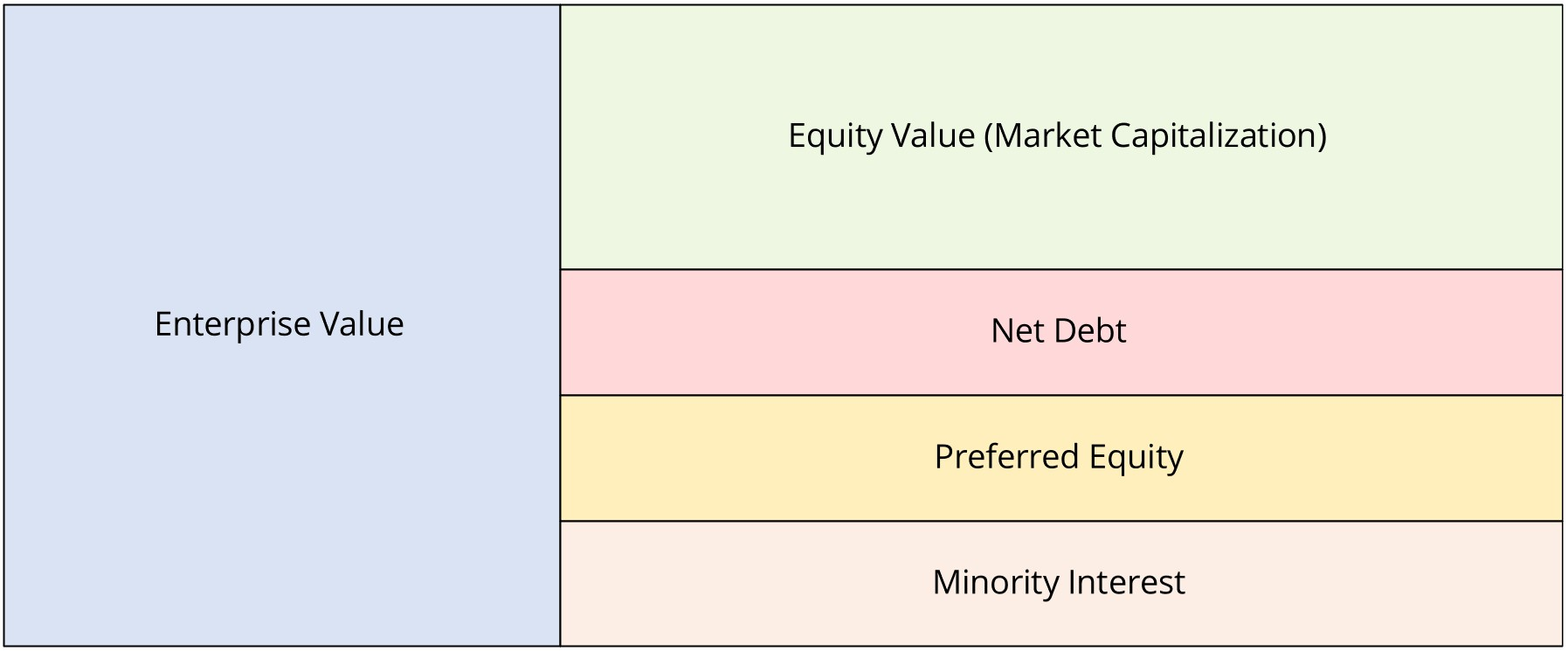###### Quick Concept Check

Q. If a company decides to raise new capital by issuing debt, does its enterprise value increase?

Contrary to a common misconception, enterprise value does not increase.

The reason is that the increase in enterprise value from the debt issuance is offset by the increase in cash, since the formula to calculate enterprise value adds net debt (i.e. plus total debt, minus cash)

## Equity Value Calculator

We’ll now move on to a modeling exercise, which you can access by filling out the form below.#### Get the Excel Template!Submitting...

## Equity Value Calculation Example

Suppose we’re tasked with calculating the equity value of Tesla (TSLA) as of the present date (09/24/2023) to compare with the “The Big 3” automakers.

In the automotive industry, “The Big Three” – Ford Motors (F), General Motors (GM), and Stellantis (STLA) – are the top three automakers in the U.S. with the greatest market share.

After compiling the current stock price and diluted shares outstanding of each company from their most recent filings, we can multiply the two figures to determine their respective equity values.

For example, Tesla’s market share is approximately \$851 billion based on our calculation.

• Equity Value – Tesla (TSLA) = \$244.88 × 3,475 million = \$851 billion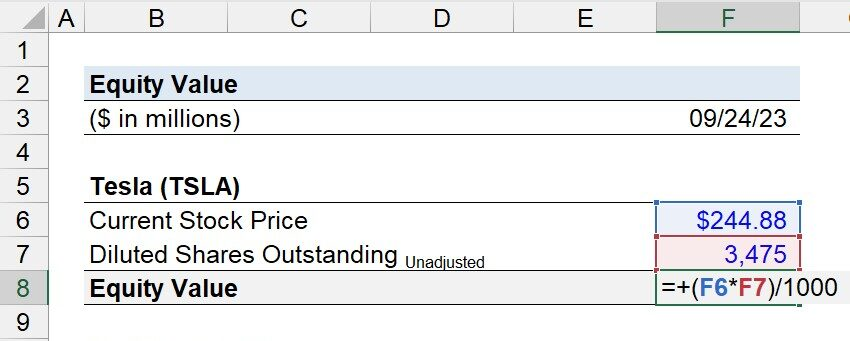Note: The diluted share count figures we’re using throughout the exercise are not fully adjusted for dilutive securities, for simplicity.

The actual share count figures – if determined using the Treasury Stock Method (TSM) – will be different, but the point intended to be illustrated here remains.

Since we’ve calculated the equity value of Tesla (TSLA), we’ll now repeat the process for Ford (F), General Motors (GM), and Stellantis (STLA).

• Equity Value – Ford (F) = \$12.43 × 4,035 million = \$50 billion
• Equity Value – General Motors (GM) = \$32.58 × 1,389 million = \$45 billion
• Equity Value – Stellantis (STLA) = \$19.35 × 3,164 million = \$61 billion
• Total Equity Value – “The Big Three” = \$50 billion + \$45 billion + \$61 billion = \$157 billion

But while the valuation of Tesla (TSLA) seems outsized compared to its industry peers, one important aspect to understand is that traditional automakers are much more dependent on debt financing.

Therefore, measuring the enterprise value (TEV) of each company as part of the analysis could portray a different picture and is much more convoluted than the oversimplified takeaway that Tesla is worth more than 5x the top automakers in the U.S. combined.

Generally speaking, the differential between the equity value and enterprise value of a company tends to increase the greater its market share, and the longer the company is positioned as a market leader (i.e. with a track record of profitability and strong free cash flow), as debt financing becomes more readily available and “cheaper” for such borrowers with less credit risk.

In conclusion, the equity value of Tesla (TSLA) is \$851 billion, whereas “The Big Three” is \$157 billion, reflecting the market’s positive sentiment on the potential upside of Tesla, where the valuation is far more comparable to that of a high-growth tech company than a traditional automotive company.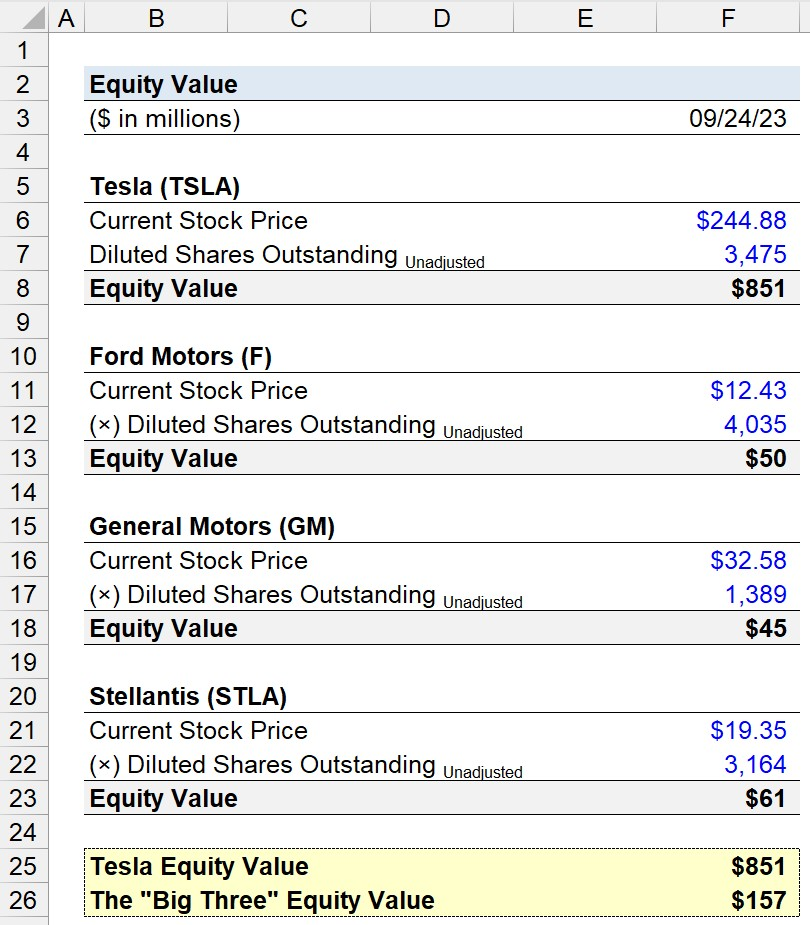## Equity Value Example: Apple (NASDAQ: AAPL)

For a real-world example, the equity value (or market capitalization) of Apple (NASDAQ: AAPL) is around \$2.7+ trillion in the fall of 2023, which is substantially higher than the \$60.3 billion in shareholders’ equity recorded on its latest 10-Q balance sheet.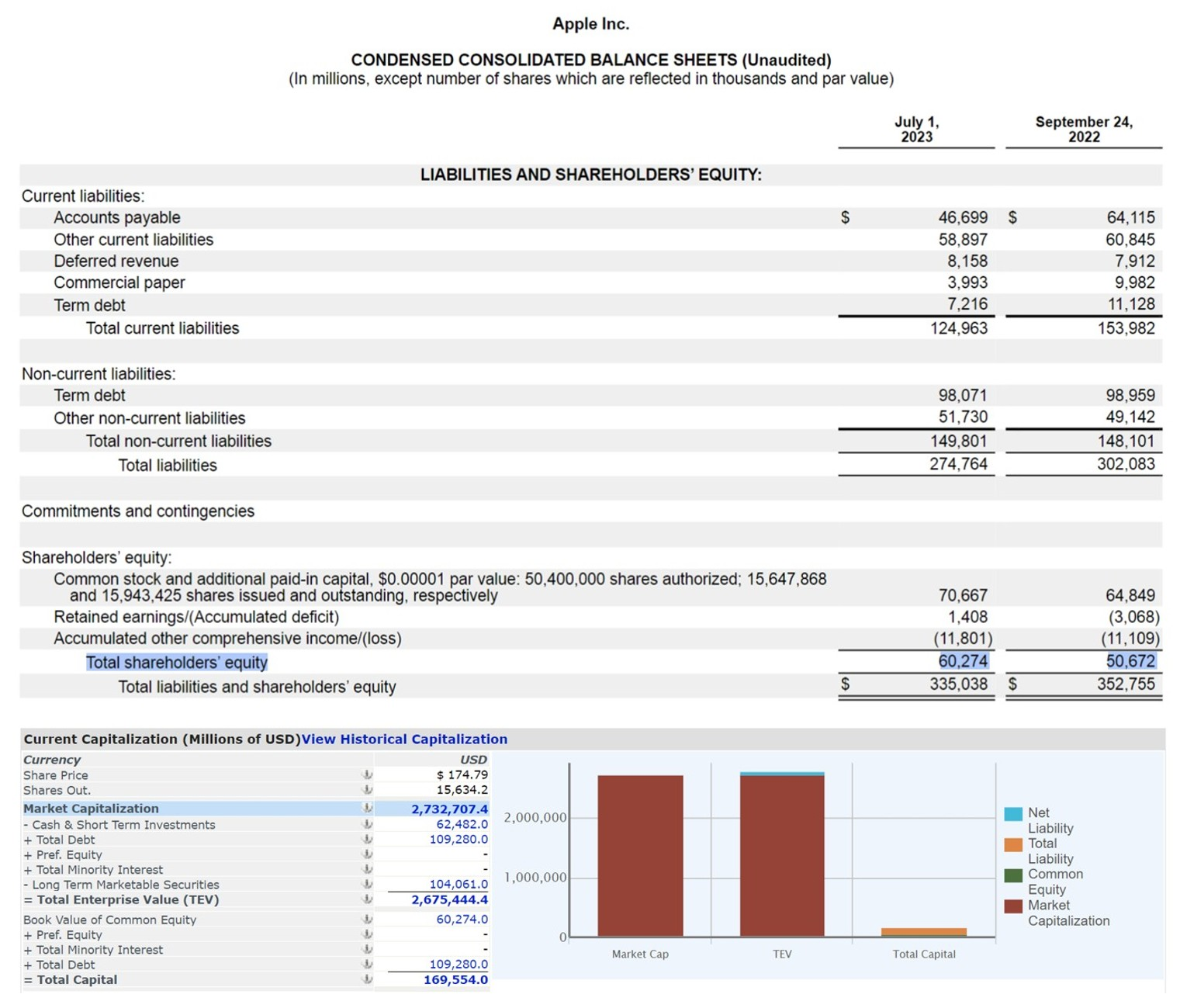Apple, Inc. Equity Value vs. Shareholders’ Equity (Source: Capital IQ; AAPL 10-Q)

Note: Capital IQ calculates the market capitalization of Apple (AAPL) using basic shares outstanding, so the actual figure is higher if adjusted for potentially dilutive securities.

### The Wharton Online and Wall Street Prep Private Equity Certificate Program

Level up your career with the world's most recognized private equity investing program. Enrollment is open for the Jan. 29 - Mar. 25 cohort.